Home / Electrical MCQs / Three Phase AC Circuits MCQs with Answers

# Three Phase AC Circuits MCQs with Answers

Want create site? Find Free WordPress Themes and plugins.

1. A three-phase generating system has an efficiency of around:

 A. 96%
 B. 90%
 C. 66%
 D. 50%

2. The windings of a three-phase alternator are separated by:

 A. 90°E
 B. 120º E
 C. 180°E
 D. 360°E

3. Figure shows the stator winding arrangement of a 4 pole, three phase alternator: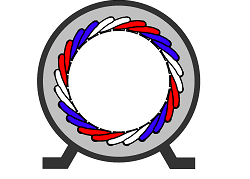The alternator has a 24-slot stator and is wound with 24 coils in sets of:

 A. 4 coils per pole per phase
 B. 3 coils per pole per phase
 C. 2 coils per pole per phase
 D. 1 coil per pole per phase

4. One of advantages of a three-phase system over a single phase system is that the power is more constant so that the torque of a rotating machine is more constant. The more constant torque results in:

 A. much more vibration from the machine
 B. no vibration from the machine
 C. excessive load current from the machine
 D. much less vibration from the machine

5. Figure shows the waveform for a three-phase AC supply: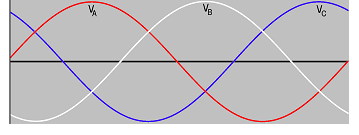The displacement between the voltage is:

 A. 120°E
 B. 60°E
 C. 90°E
 D. 30°E

6. In a three-phase system, where the three voltage sources are, connected to feed a three-phase load, the phase sequence is important for rotating machinery, because the:

 A. direction of current flow will increase
 B. direction of rotation will be affected
 C. machinery could draw excessive load current

7. One method of forming a three-phase system is to connect the three similar ends of the windings together at one point. This type of connection is called a:

 A. parallel connection
 B. delta connection
 C. star connection
 D. mesh connection

8.
The three-phase winding shown in figure have been connected in the:

 A. delta configuration
 B. double series configuration
 C. parallel configuration
 D. star configuration

9.
The three-phase winding shown in figure has:

 A. A B C rotation
 B. BAC rotation
 C. CAB rotation
 D. ACB rotation

10. In a three-phase star-connected system the line current equals:

 A. Ö3 x the phase current
 B. the phase current
 C. 1.732 x the phase current
 D. the line voltage

11. In a three-phase star-connected system the line voltage equals:

 A. the phase voltage
 B. the phase voltage / 1.732
 C. Ö3 x the phase voltage
 D. the line current

12. Figure shows one method of determining the line voltage of a three-phase star connected system: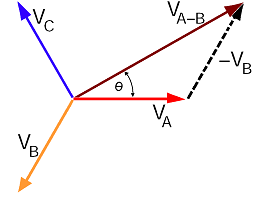In the diagram, the line voltage phasor VA-B is equal to:

 A. VA + VB
 B. Ö2 VA
 C. 1.414 x VA
 D. Ö3 VA

13. In a star connected three-phase system, the line voltages:

 A. lead the phase voltages by 30°E
 B. lag the phase voltages by 30°E
 C. lead the phase voltages by 60°E
 D. lag the phase voltages by 60°E

14. Figure shows the phasor diagram method of determining the line voltage of a three-phase star connected system: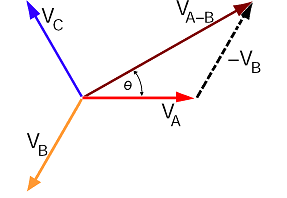In the diagram, the line voltage phasor VA-B

 A. lags the phase voltage VA by 60°E
 B. leads the phase voltage VA by 30°E
 C. leads the phase voltage VA by 60°E
 D. lags the phase voltage VA by 30°E

15. Figure shows the phasor diagram of a three-phase star connected system in which phase “C” phase has mistakenly been connected with the connections reversed:The waveforms of the three phase voltages then have a displacement of 120º E between A and B, and:

 A. 90º E between A and C and also between C and B
 B. 180º E between A and C and also between C and B
 C. 60º E between A and C and also between C and B
 D. 120º E between A and C and also between C and B

16. One method of forming a three-phase system is to connect the dissimilar similar ends of the windings together. This type of connection is called a:

 A. parallel connection
 B. wye connection
 C. star connection
 D. delta connection

17.
The three-phase winding shown in figure have been connected in the:

 A. delta configuration
 B. double series configuration
 C. parallel configuration
 D. star configuration

18. In a three-phase delta-connected system the line current equals:

 A. the phase current
 B. Ö3 x the phase current
 C. 1.732 x the phase voltage
 D. the line voltage

19. In a three-phase delta-connected system the line voltage equals:

 A. Ö3 x the phase voltage
 B. the phase voltage / 1.732
 C. the phase voltage
 D. the line current

20. Figure shows one a Delta connected 400 V three-phase system in which one phase has been mistakenly connected with reversed connections: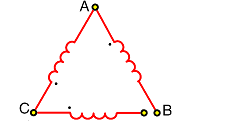In the diagram, the voltage between the open connections will be:

 A. 230 V
 B. 400 V
 C. 415 V
 D. 800 V

21. Figure shows a connection diagram for a three-phase delta connected load: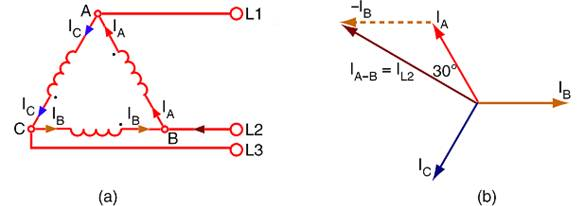The current in line 2, equals the:

 A. phasor difference of phase currents IA and IB
 B. phasor sum of phase currents IA and IB
 C. arithmetic sum of phase currents IA and IB
 D. arithmetic difference of phase currents IA and IB

22. Figure shows a phasor diagram for determining the line current in a three-phase delta connected load: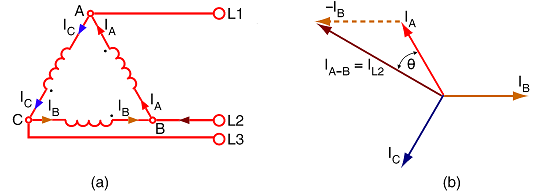In the diagram, the line current phasor IL2

 A. leads the phase current IA by 60°E
 B. leads the phase current IA by 30°E
 C. lags the phase voltage VA by 60°E
 D. lags the phase voltage VA by 30°E

23. Electrical power can be transmitted using low voltage and high currents. Higher currents result in:

 A. lower transmission losses
 B. very efficient transmission
 C. higher voltage outputs
 D. higher transmission losses

24. In economic terms, the higher the voltage used for power transmission systems, the:

 A. lower the cost of installing and maintaining the transmission lines
 B. higher the cost of installing and maintaining the transmission lines
 C. higher the cost of the current along the transmission lines
 D. lower the cost of installing underground transmission lines

25.
Transmission system voltages are far higher than the voltages required by the average consumer, therefore the voltage is:

 A. stepped up to a suitable value using transformers
 B. stepped down to a suitable value using transformers
 C. kept the same right through to the customer’s circuits
 D. converted to DC for the customer’s machines

26.
The distribution system shown in figure is a:

 A. switched wiring electrical reticulation system
 B. sub-transmission electrical receiving system
 C. single wire earth return distribution system
 D. sixteen-kilovolt wiring earthed resister system

27. Figure shows a 4-wire distribution system with the star point of the phase windings connected to earth: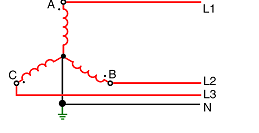One advantage of this system is that:

 A. only one voltage is available to the consumer
 B. the neutral conductor is not used by the consumer
 C. the active conductors are connected to the earth
 D. two voltages are available to the consumer

28. For a balanced load, the current in a neutral conductor of a three-phase, four-wire system is equal to:

 A. zero
 B. the current in any phase
 C. the arithmetic sum of the phase currents
 D. the phasor difference of any two line currents

29. For any type of load, the current in a neutral conductor of any three-phase, four-wire system is equal to:

 A. the phaser difference of the line currents
 B. minus the phasor sum of the line currents
 C. minus the arithmetic sum of the line currents
 D. the arithmetic difference of the line currents

30. The currents in the lines of a three-phase four-wire system are:
A phase—8 amperes at unity power factor
B phase—10 amperes at 0.866 leading power factor
C phase—10 amperes at 0.866 lagging power factor.
In this case the current flowing in the neutral conductor will be approximately:

 A. 28 A
 B. 10 A
 C. 8 A
 D. 12 A

31. Figure shows the readings obtained when checking the voltages of a faulty 230/400 V three-phase four-wire system: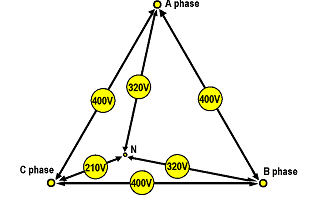The most likely cause of the fault will be a:

 A. faulty three-phase alternator
 B. faulty three-phase pole-top transformer
 C. high resistance load on one phase
 D. broken neutral conductor

32. The power consumed by a three-phase load can be determined using the formula:

 A. P = √3 V I cos ø
 B. P = 3 V I cos ø
 C. P = √3 V I
 D. P = √3 V I sin ø

33. A three-phase 400 V motor draws 10 A at 0.8 lagging power factor. The power is consumed by this motor will be approximately:

 A. 6.93 kW
 B. 5.54 kW
 C. 4.00 kW
 D. 3.20 kW

34. Figure shows the one wattmeter method of measuring three-phase power on a three-phase four-wire system: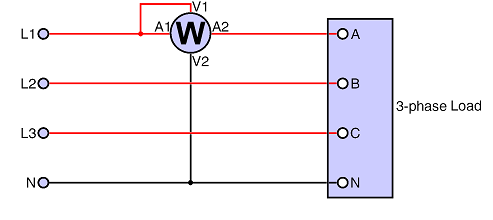One advantage of this method is that:

 A. only one reading is needed
 B. it is suitable for fluctuating loads
 C. only one wattmeter is required
 D. a neutral connection is not required

35. Figure shows the two-wattmeter method of measuring power in a three-phase three-wire system: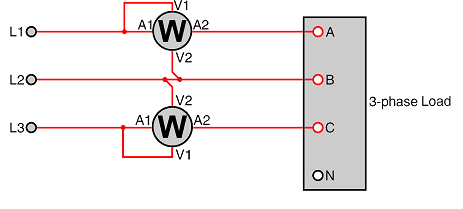One advantage of using this method is that:

 A. a neutral conductor is available for the meters
 B. the meters only need to measure the phase voltages
 C. three-terminal wattmeters can be used
 D. the power factor can be obtained for balanced loads

36. The power consumed by a three-phase motor has been measured using the two-wattmeter method. At full load, the two wattmeters gave readings of 6 kW and 3 kW. At this load, the power factor of the motor will be:

 A. 0.866 lagging
 C. 0.189 lagging

37. Figure shows the three-wattmeter method of measuring three-phase power in a three-phase four-wire system: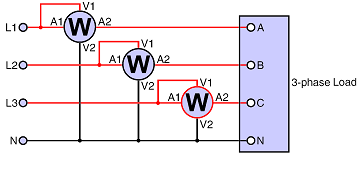To find the total power, the following calculation must be made using the readings: PTOTAL

 A. W1 + W2 / W3
 B. W1 + W2 + W3
 C. W1 – W2. / W1 + W3
 D. W1 / W2 + W3

38. Figure shows the three-wattmeter method of measuring three-phase power in a three-phase four-wire system.This method is suitable for:

 D. measuring the power factor with balanced loads

39. One advantage of using three-phase digital wattmeters is as well as the power, they can show the value of such things as:

 A. the current in each phase
 B. the VAR value for each phase
 C. frequency and total harmonic distortion
 D. all of the given answers

40. In power stations operating under normal conditions, the values of voltage and current are generally too high and unwieldy to be used directly with portable instruments. To avoid these high-energy circuits when measuring voltage, current and power, in these cases:

 A. potential transformers and current transformers must be used
 B. only high voltage ammeter and voltmeters must be used
 C. digital voltmeters can be used with a high voltage extension arm
 D. 275 kV ammeters and voltmeters must be used for 11 kV circuits

Did you find apk for android? You can find new Free Android Games and apps.